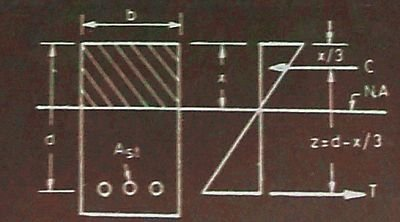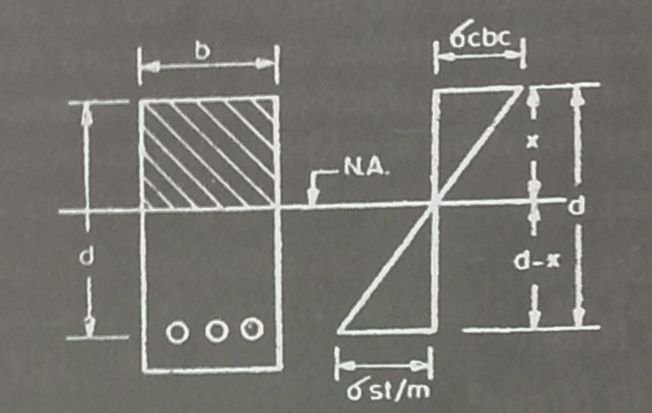## Moment of Resistance | Design of Singly reinforced Sections

#### Moment of Resistance | Guide to design of Singly reinforced Sections

For the design of Singly reinforced Sections article series, we have covered the following:

Now we will move on with our discussion on “Moment of resistance” and derive the formula for Moment of resistance for balanced section, under-reinforced section and over reinforced section.

The moment of resistance of the concrete section is the moment of couple formed by the total tensile force (T) in the steel acting at the centre of gravity of reinforcement and the total compressive force (C) in the concrete acting at the centre of gravity (c.g.) of the compressive stress diagram. The moment of resistance is denoted by M.

The distance between the resultant compressive force (C) and tensile force (T) is called the lever arm, and is denoted by z.Moment of resistance | Singly reinforced Section

From the diagram above, it is clear that the intensity of compressive stress varies from maximum at the top to zero at the neutral axis.

Therefore, centre of gravity of the compressive force is at a distance x/3 from the top edge of the section.

Therefore, z = d-x/3

Read more

## Solved Numericals for Singly reinforced Sections | Design Method 2

#### Design of Singly reinforced Sections | Method 2

In our article series for Singly reinforced sections, we have covered the following:

#### Numerical Problem

Find the position of the neutral axis of a reinforced concrete beam 150mm wide and 400mm deep (effective). Area of tensile steel is 804mm2. (modular ratio = m = 18.66)

#### Given that:

b = breadth of a rectangular beam = 150mm

d = effective depth of a beam = 400mm

Ast = cross-sectional area of steel in tension = 804mm2

x = depth of neutral axis below the compression edge

m = modular ratio = 18.66

Taking moments of area of compression and tension sides about neutral axis,

bx.x/2 = mAst (d – x)

Read more

## Design of Singly reinforced sections | Design Method 2

#### Guide to design of Singly reinforced Sections

For “Singly reinforced sections” article series, we have covered the following:

Now we will move on with our discussion on “2nd Design method” for the design of Singly reinforced Sections.
We will follow a simple three-step procedure for the design of singly reinforced sections.

#### Step One:

Given that:

• Dimensions of section (b and d)
• Area of tensile steel (Ast)
• Modular ratio (m)Stress-strain diagram

From the figure above, we can see that the neutral axis is situated at the centre of gravity of a given section. Therefore, the moments of area on either side are equal.

Read more

## Design Methods for Singly reinforced Sections

#### Singly reinforced sections | Design of RCC structures

Earlier we discussed some basic terms in reference to singly reinforced sections design. It is important that you are thorough with the basic definitions and have complete understanding of stresses in concrete and steel. You should also possess the knowledge of reinforcement and terminology of beams which includes understanding singly reinforced beam, doubly reinforced beam, under reinforced beam, over reinforced beam and balanced reinforced beam.

There are two methods for the design of singly reinforced sections. In this article we will discuss the first method of singly reinforced section in a stepwise manner. The discussion will include the method for determining the value of neutral axis followed by a formula for the area of steel calculations.

Let,

b = breadth of a rectangular beam

d = effective depth of a beam

x = depth of neutral axis below the compression edge

Ast = cross-sectional area of steel in tension

σcbc = permissible compressive stress in concrete in bending

σst = permissible stress in steel

m = modular ratio

Neutral axis

Neutral axis is denoted as NA.

There are two methods for determining the neutral axis depending on the data given.Stress strain diagram

In this article, we will discuss the first method followed by a couple of numericals for your understanding and then move on to the second method.

Read more